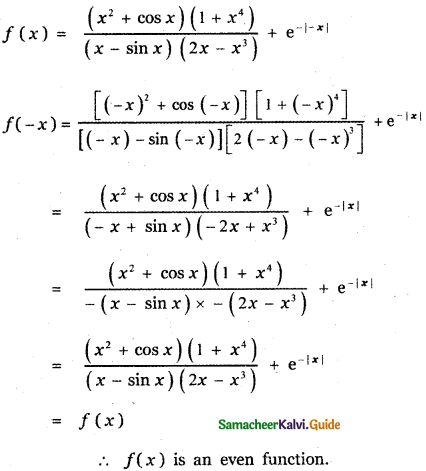Tamilnadu State Board New Syllabus Samacheer Kalvi 11th Maths Guide Pdf Chapter 1 Sets, Relations and Functions Ex 1.5 Text Book Back Questions and Answers, Notes.

## Tamilnadu Samacheer Kalvi 11th Maths Solutions Chapter 1 Sets, Relations and Functions Ex 1.5

Choose the correct or the most suitable answer.

Question 1.
If A = {(x,y): y = ex, x ∈ R} and B = {(x, y): y = e-x, x ∈ R},then n(A ∩ B) is
(1) Infinity
(2) 0
(3) 1
(4) 2
(3) 1Explaination:
Given
A = {(x,y): y = ex, x ∈ R}
B = {(x,y): y = e-x, x ∈ R}
Consider the curve y = ex
When x = 0 ⇒ y = e-0 = 1
When x = ∞ ⇒ y = e-∞ = ∞
When x = -∞ ⇒ y = e = 0

Consider the curve y = e-x.
When x = 0 ⇒ y = e-0 = 1
When x = ∞ ⇒ y = e-∞ = 0
When x = -∞ ⇒ y = e = ∞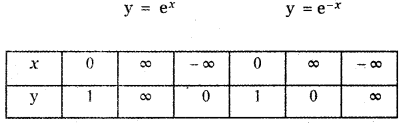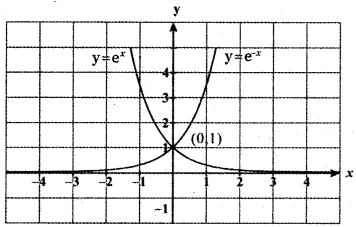(0, 1) is the only point common to y = e<sup.x and y = e-x
∴ A ∩ B = {(0,1)} ⇒ n (A ∩ B ) = 1Question 2.
If A = { ( x, y): y = sin x, x ∈ R } and B = { ( x, y): y = cos x, x ∈ R} then A ∩ B contains
(1) no element
(2) infinitely many elements
(3) only one element
(4) cannot be determined
(2) infinitely many elements

Explaination:
Given A = { (x, y): y = sin x, x ∈ R}
B = {(x, y): y = cos x, x ∈ R }
Consider the equations y = sin x and y = cos x
sin x = cos x ⇒ $$\frac{\sin x}{\cos x}$$ = 1
tan x = 1 ⇒ x = nπ +  for n ∈ z
There are infinite number of common points for the sets A and B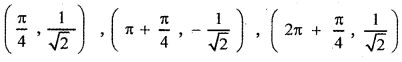Question 3.
The relation R defined on a set A = {0, -1, 1, 2} by x R y if |x2 + y2| ≤ 2,
then which one of the following is true.
(1) R = {(0,0), (0,-1), (0,1), (-1,0), (-1,1), (1,2), (1,0)}
(2) R-1 = {(0, 0), (0, -1), (0, 1), (-1, 0), (1, 0)}
(3) Domain of R is {0,- 1, 1, 2}
(4) Range of R is {0, -1, 1}
(4) Range of R is {0, -1, 1}

Explaination:
A= {0, -1, 1, 2}
|x2 + y2| ≤ 2
The values of x and y can be 0, -1 or 1
So range = {0, -1, 1}Question 4.
If f(x) = |x – 2 | + |x + 2| x ∈ R then
(1)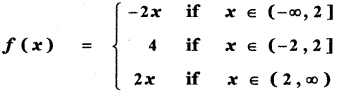(2)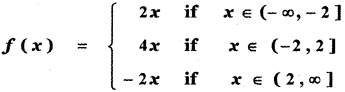(3)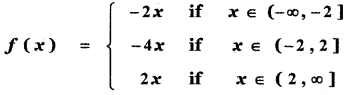(4)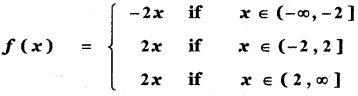(1)Explaination:
f(x) = |x – 2| + |x + 2|, x ∈ R
Divide the Real line into three intervals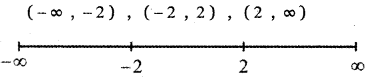In the interval (2, ∞), both the factors x – 2 and x + 2 are positive.
∴ f(x) = x – 2 + x + 2 = 2x
f(x) = 2x for all x ∈ (2, ∞)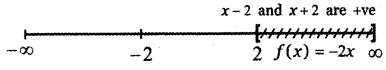In the interval (- ∞, – 2 ] both the factors x – 2 and x + 2 are negative.
∴ f(x) = – (x – 2) – (x + 2)
= – x + 2 – x – 2 = – 2x
∴ f(x) = – 2x for all x ∈ (- ∞,- 2]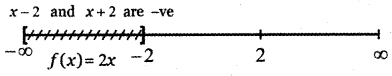In the interval (—2, 2], the factor x – 2 is negative and the factor x + 2 is positive.
∴ f(x) = – (x – 2) + (x + 2)
f(x) = – x + 2 + x + 2 = 4
Thus f(x) = 4 for all x ∈ (- 2, – 2]Question 5.
Let R be the set of all real numbers. Consider the following subsets of the plane R × R:
S = { (x, y): y = x + 1 and 0 < x < 27 and
T = {(x, y): x – y is an integer} Then which of the following is true?
(1) T is an equivalence relation but S Is not an equivalence relation
(2) Neither S nor T is an equivalence relation
(3) Both S and T are equivalence relation
(4) S is an equivalence relation but T is not an equivalence relation.
(1) T is an equivalence relation but S Is not an equivalence relation

Explanation:
(0, 1), (1, 2) it is not an equivalence relation
T is an equivalence relationQuestion 6.
Let A and B be subsets of the universal set N, the set of natural numbers. Then A’ ∪ [(A ∩ B) ∪ B’] is
(1) A
(2) A’
(3) B
(4) N
(4) N

Explaination:
Let N = {1, 2, 3, ……….. 10}
A = { 1, 2, 3, 4, 5 }
B = {6, 7, 8, 9, 10}
A’ = {6, 7, 8, 9, 10 }
B’ = { 1 , 2, 3, 4, 5 }
A ∪ B = {1, 2, 3, 4, 5} ∪ {6, 7, 8, 9, 10}
A ∪ B = {1, 2, 3, 4, 5, 6, 7, 8, 9, 10}
(A ∪ B) ∩ B’ = {1,2, 3, 4, 5, 6,7, 8, 9,10} ∩ { 1, 2, 3, 4 , 5 }
(A ∪ B) ∩ B’= {1,2, 3,4, 5}
A’ ∪ [(A ∪ B ) ∩ B’] = { 6, 7, 8, 9 , 10 } ∪ {1, 2, 3, 4, 5 }
= {1, 2, 3, 4,5, 6, 7, 8, 9, 10}
A’ ∪ [(A ∪ B) ∩ B’] = NQuestion 7.
The number of students who take both the subjects Mathematics and Chemistry is 70. This represents 10% of the enrollment in Mathematics and 14% of the enrollment in Chemistry. The number of students takes atleast one of these two subjects, is
(1) 1120
(2) 1130
(3) 1100
(4) Insufficient data
(2) 1130

Explanation:
n(M ∪ C) = n(M) + n(C) – n(M ∩ C)
= 700 + 500 – 70
= 1130Question 8.
If n[ (A × B) n (A × C) ] = 8 and n(B ∩ C) = 2 then n(A) is
(1) 6
(2) 4
(3) 8
(4) 16
(2) 4

Explaination:
Given n[(A × B) n (A × C)] = 8
n(B ∩ C) = 2
n[(A × B) ∩ (A × C)] = 8
A × (B ∩ C) = (A × B) ∩ (A × C) ]
⇒ n [A × (B ∩ C)] = 8
⇒ n(A) . n (B ∩ C) = 8
⇒ n(A). 2 = 8
⇒ n(A) = $$\frac{8}{2}$$ = 4

Question 9.
If n(A) = 2 and n(B ∪ C) = 3,then n[(A × B) ∪ (A × C)] is
(1) 23
(2) 32
(3) 6
(4) 5
(3) 6

Explaination:
n[(A × B) ∪ (A × C)] = n[ A × (B ∪ C)] = n(A) × n(B ∪ C)
= 2 × 3
= 6Question 10.
If two sets A and B have 17 elements in common, then the number of elements common to the set A × B and B × A is
(1) 217
(2) 172
(3) 34
(4) Insufficient data
(2) 172

Explanation:
If two sets A and B have 17 elements in common, then the number of elements common to the set A × B
and B × A is 172

Question 11.
For non-empty sets A and B, if A ⊂ B then (A × B) ∩ (B × A) is equal to
(1) A ∩ B
(2) A × A
(3) B × B
(4) None of these
(2) A × A

Explanation:
When A ⊂ B, (A × B) ∩ (B × A) = A × AQuestion 12.
The number of relations on a set containing 3 elements is
(1) 9
(2) 81
(3) 512
(4) 1024
(3) 512

Explanation:
The number of relations on a set containing n elements is 2n2. Here n = 3
∴ Required number = 232 = 29
= 512

Question 13.
Let R be the universal relation on a set X with more than one element. Then R is
(1) not reflexive
(2) not symmetric
(3) transitive
(4) none of the above
(3) transitive

Explanation:
Given R is a universal relation on the set X.
The universal relation is always an equivalence relation.
R is reflexive, symmetric, and transitive.Question 14.
Let X = { 1, 2, 3, 4 } and R = { ( 1, 1 ), (1, 2), (1, 3), (2, 2), (3, 3), (2, 1), (3, 1), (1, 4), ( 4, 1) } . Then R is
(1) Reflexive
(2) Symmetric
(3) Transitive
(4) Equivalence
(2) Symmetric

Explanation:
(4, 4} ∉ R ⇒ R is not reflexive
(1, 4), (4, 1) ∈ R ⇒ R is symmetric
(1, 4), (4, 1) ∈ R but (4, 4) ∉ R
So R is not transitive

Question 15.
The range of the function $$\frac{1}{1-2 \sin x}$$ is
(1) (- ∞, – 1) ∪ ($$\frac{1}{3}$$, ∞)
(2) (-1, $$\frac{1}{3}$$)
(3) [-1, $$\frac{1}{3}$$]
(4) (- ∞, – 1] ∪ [$$\frac{1}{3}$$, ∞)
(4) (- ∞, – 1] ∪ [$$\frac{1}{3}$$, ∞)

Explaination:Question 16.
The range of the function
f(x) = |[x] – x|, x ∈ R is
(1) [0, 1]
(2) [0, ∞)
(3) [0, 1)
(4) (0, 1)
(3) [0, 1)

Explaination:
f(x) = |[x] – x|
When x = 1 ,
we have [x] =  = 1
f(1) = |1 – 1| = o

When x = 1.5
we have [x} = [1.5] = 1
f(1.5) = |1 – 1.5| = |- 0.5| = 0.5

When x = 2.5
we have [x] = [2.5] = 2
f (2.5) = |2 – 2.5| = |- 0.5| = 0.5

When x = – 2.5
we have [x] = [- 2.5] = – 3
f (-2.5) = |- 3 – (-2.5| = |- 3 + 0.5| = |- 0.5| = 0.5
∴ Range of f(x) = |[x] – x| is [0, 1)Question 17.
The rule f(x) = x2 is a bijection if the domain and the co – domain are given by
(1) R, R
(2) R,(0, ∞)
(3) (0, ∞)R
(4) [0, ∞), [0, ∞)
(4) [0, ∞), [0, ∞)

Explaination:
Let x ∈ R, then x can be negative or zero or positive.
Given f(x) = x2
The image of x under f is always positive since x2 is positive for x = 1 and x = – 1 ∈ R
f(1) = 12 = 1
f(-1) = (-1)2 = 1
∴ 1, – 1 have the same image
∴ f is not one – one if the domain is R.
Suppose the domain is [0, ∞) then f is one – one and onto.
Domain = [0, ∞)
Co-domain = [0, ∞)Question 18.
The number of constant functions from a set containing m elements to a set containing n elements is
(1) mn
(2) m
(3) n
(4) m + n
(3) n

Explanation:
Let A be a set having m elements and B be a set having n elements.
When all the elements of A mapped onto the first element of B we get the first constant function. When all the elements of A mapped onto the second element of B we get the second constant function.When all the elements of A mapped on to the nth element of B, we get the nth constant function.
∴ The number of constant functions possible is n.Question 19.
The function f:[0, 2π] → [- 1, 1] defined by f(x) = sin x is
(1) one to one
(2) onto
(3) bijection
(4) cannot be defined
(2) onto

Explaination:
f : [0, 2π] → [- 1, 1]
Defined by f (x) = sin x
f(0) = sin 0 = 0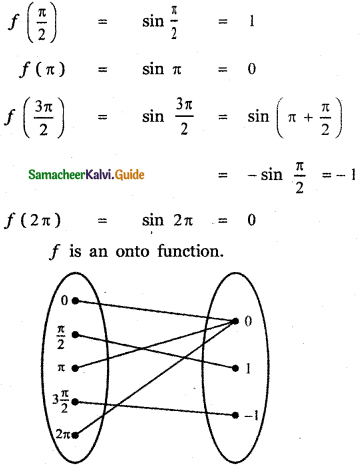Question 20.
If the function f : [-3, 3] → S defined by f(x ) = x2 is onto, then S is
(1) [-9, 9]
(2) R
(3) [-3, 3]
(4) [0, 9]
(4) [0, 9]

Explaination:
f: [-3, 3] → S defined by f(x) = x2
f(-3) = (-3)2 = 9
f(0) = 02 = o
f(3) = 32 = 9
∴ S = [0, 9]

Question 21.
Let X = {1, 2, 3, 4}, Y = { a, b , c, d } and f = {(1, a), (4, b), (2, c), (3, d), (2, d)}. Then f is
(1) an one – to – one function
(2) an onto function
(3) a function which is not one to one
(4) not a function
(4) not a function

Explaination:
X = {1, 2, 3, 4}, Y = {a, b, c, d>
f = {(1, a), (4, b), (2, c), (3, d), (2, d)}f is not a function since 2 ∈ X has two images c and d.Question 22.
The inverse of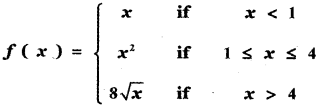(1)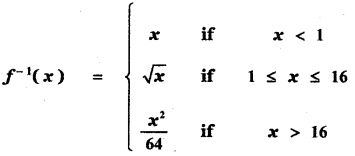(2)(3)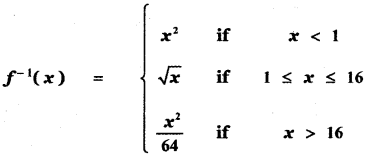(4)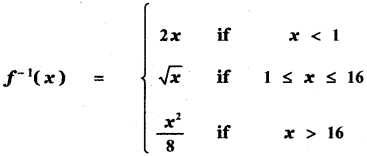(1)Explaination: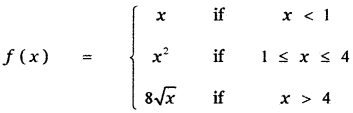Let f(x) = x if x < 1 —— (1)
Put y = x then
(1) ⇒ f(x) = y
⇒ x = f-1(y) if y < 1
⇒ y = f-1(y) if y < 1
⇒ f-1(x) = x if x < 1
Let f(x) = x2 if 1 ≤ x ≤ 4 —– (2)
Put y = x2 ⇒ x = √y, if 1 ≤ y ≤ 16
then (2) ⇒ f(x) = y
⇒ x = f-1(y) if 1 ≤ y ≤ 16
⇒ √(y) = f-1(y) if 1 ≤ y ≤ 16
⇒ √x = f-1(y) if 1 ≤ x ≤ 16
Let f(x) = 8√x if x > 4 ———– (3)
Put y = 8√x ⇒ y2 = 64 x
⇒ x = $$\frac{y^{2}}{64}$$ if y>16
then (3) ⇒ f(x) = y
⇒ x = f-1(y) if y > 16
⇒ √y = f-1(y) if y > 16
⇒ $$\frac{y^{2}}{64}$$ = f-1(x) if x > 16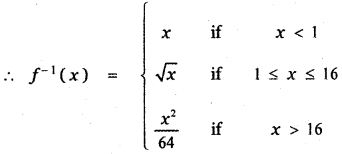Question 23.
Let f: R → R be defined by f(x) = 1 – |x|. Then the range of f is
(1) R
(2) (1, ∞)
(3) (-1, ∞)
(4) (- ∞, 1)
(4) (- ∞, 1)

Explaination:
f: R ➝ R defined by
f(x) = 1 – |x|
For example,
f(1) = 1 – 1 = 0
f(8) = 1 – 8 = -1
f(-9) = 1 – 9 = -8
f(-0.2) = 1 – 0.2 = 0.8
so range = (-∞, 1]

Question 24.
The function f : R → R be defined by f(x) = sin x + cos x is
(1) an odd function
(2) neither an odd function nor an even function
(3) an even function
(4) both odd function and even function
(2) neither an odd function nor an even function

Explaination:
f : R → R is defined by f(x) = sin x + cos x
f(-x) = sin (-x) + cos (-x) = -sin x + cos x ≠ f (x)
If f(-x) = -f(x) then f(x) is an odd function.
If f(-x) = f(x) then f(x) is an even function.
∴ f (x) is neither odd function nor an even function.Question 25.
The function f : R → R be defined by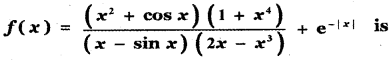(1) an odd function
(2) neither an odd function nor an even function
(3) an even function
(4) both odd function and even function.